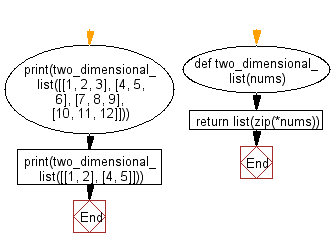﻿ Python: Create a two-dimensional list from given list of lists - w3resource# Python: Create a two-dimensional list from given list of lists

## Python List: Exercise - 264 with Solution

Write a Python program to create a two-dimensional list from given list of lists.

• Use *nums to get the provided list as tuples.
• Use zip() in combination with list() to create the transpose of the given two-dimensional list.

Sample Solution:

Python Code:

``````def two_dimensional_list(nums):
return list(zip(*nums))
print(two_dimensional_list([[1, 2, 3], [4, 5, 6], [7, 8, 9], [10, 11, 12]]))
print(two_dimensional_list([[1, 2], [4, 5]]))
```
```

Sample Output:

```[(1, 4, 7, 10), (2, 5, 8, 11), (3, 6, 9, 12)]
[(1, 4), (2, 5)]
```

Flowchart:## Visualize Python code execution:

The following tool visualize what the computer is doing step-by-step as it executes the said program:

Python Code Editor:

Have another way to solve this solution? Contribute your code (and comments) through Disqus.

What is the difficulty level of this exercise?

Test your Python skills with w3resource's quiz

﻿

## Python: Tips of the Day

Floor Division:

When we speak of division we normally mean (/) float division operator, this will give a precise result in float format with decimals.

For a rounded integer result there is (//) floor division operator in Python. Floor division will only give integer results that are round numbers.

```print(1000 // 300)
print(1000 / 300)```

Output:

```3
3.3333333333333335```